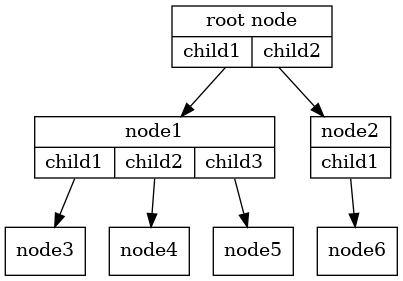# 6.3. Vocabulary and Definitions¶

Now that we have looked at examples of trees, we will formally define a tree and its components.

Node

A node is a fundamental part of a tree. It can have a name, which we call the key. A node may also have additional information. We call this additional information the value or payload. While the payload information is not central to many tree algorithms, it is often critical in applications that make use of trees.

Edge

An edge is another fundamental part of a tree. An edge connects two nodes to show that there is a relationship between them. Every node (except the root) is connected by exactly one incoming edge from another node. Each node may have several outgoing edges.

Root

The root of the tree is the only node in the tree that has no incoming edges. In Figure 2, / is the root of the tree.

Path

A path is an ordered list of nodes that are connected by edges, for example, Mammalia $$\rightarrow$$ Carnivora $$\rightarrow$$ Felidae $$\rightarrow$$ Felis $$\rightarrow$$ catus is a path.

Children

The set of nodes that have incoming edges from the same node are said to be the children of that node. In Figure 2, nodes log/, spool/, and yp/ are the children of node var/.

Parent

A node is the parent of all the nodes it connects to with outgoing edges. In Figure 2 the node var/ is the parent of nodes log/, spool/, and yp/.

Sibling

Nodes in the tree that are children of the same parent are said to be siblings. The nodes etc/ and usr/ are siblings in the file system tree shown in Figure 2.

Subtree

A subtree is a set of nodes and edges comprised of a parent and all the descendants of that parent.

Leaf Node

A leaf node is a node that has no children. For example, Human and Chimpanzee are leaf nodes in Figure 1.

Level

The level of a node $$n$$ is the number of edges on the path from the root node to $$n$$. For example, the level of the Felis node in Figure 1 is five. By definition, the level of the root node is zero.

Height

The height of a tree is equal to the maximum level of any node in the tree. The height of the tree in Figure 2 is two.

With the basic vocabulary now defined, we can move on to a formal definition of a tree. In fact, we will provide two definitions of a tree. One definition involves nodes and edges. The second definition, which will prove to be very useful, is a recursive definition.

Definition One: A tree consists of a set of nodes and a set of edges that connect pairs of nodes. A tree has the following properties:

• One node of the tree is designated as the root node.

• Every node $$n$$, except the root node, is connected by an edge from exactly one other node $$p$$, where $$p$$ is the parent of $$n$$.

• A unique path traverses from the root to each node.

• If each node in the tree has a maximum of two children, we say that the tree is a binary tree.

Figure 3 illustrates a tree that fits definition one. The arrowheads on the edges indicate the direction of the connection.Figure 3: A Tree Consisting of a Set of Nodes and Edges¶

Definition Two: A tree is either empty or consists of a root and zero or more subtrees, each of which is also a tree. The root of each subtree is connected to the root of the parent tree by an edge. Figure 4 illustrates this recursive definition of a tree. Using the recursive definition of a tree, we know that the tree in Figure 4 has at least four nodes since each of the triangles representing a subtree must have a root. It may have many more nodes than that, but we do not know unless we look deeper into the tree.